# 全基因组甲基化测序分析

## 产品介绍

DNA甲基化是重要的表观遗传学标记信息，获得全基因组范围内所有C位点的甲基化水平数据，对表观遗传学的时空特异性研究具有重要意义。Bisulfite甲基化测序是以新一代高通量测序平台为基础，结合全基因组Bisulfite处理和生物信息数据分析技术，进行低成本、高效率、高准确度的全基因组DNA甲基化水平图谱绘制。

## 分析内容流程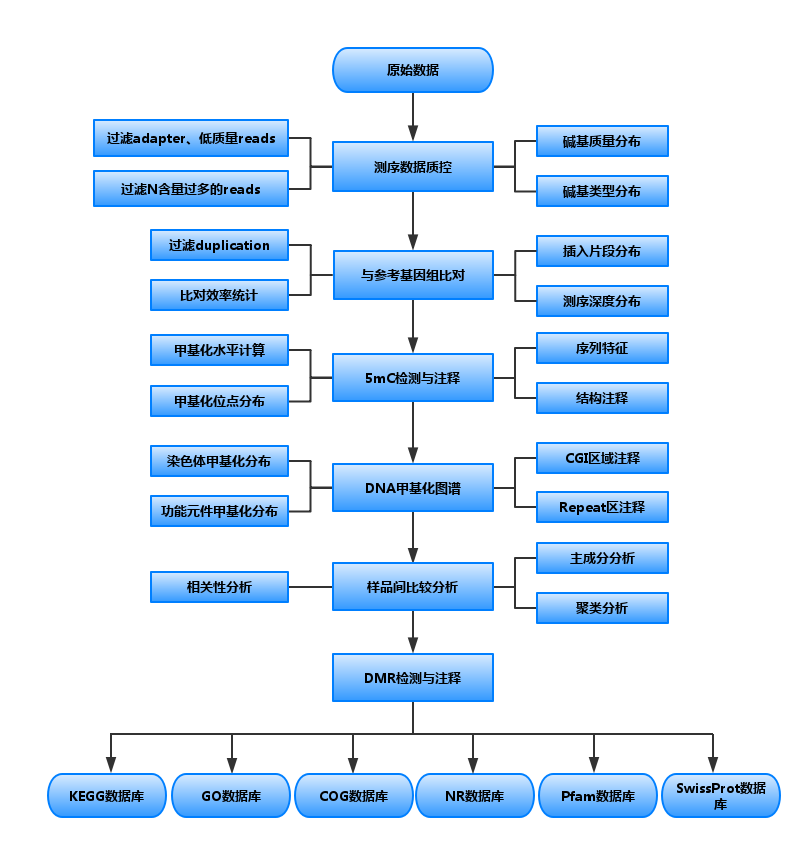## 结果展示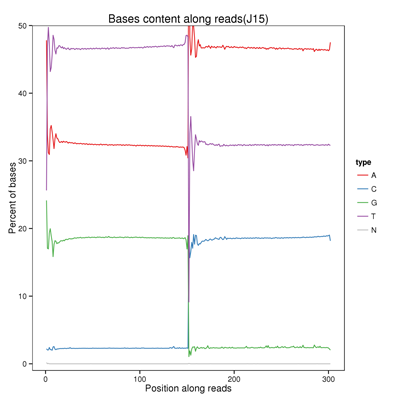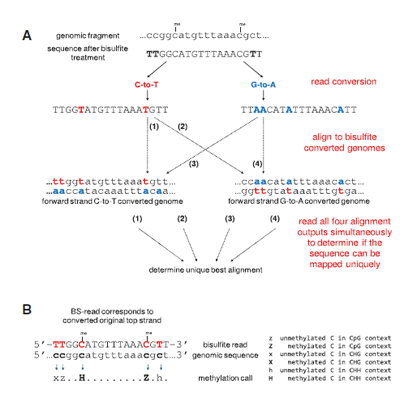5mC检测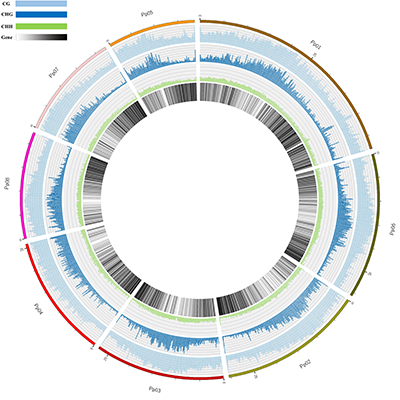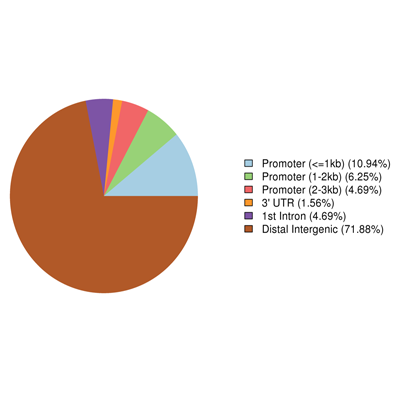DMR相关GO基因分类注释

DMR相关基因GO注释分类统计图，直观的反映出在生物过程（biological process）、细胞组分（cellular component）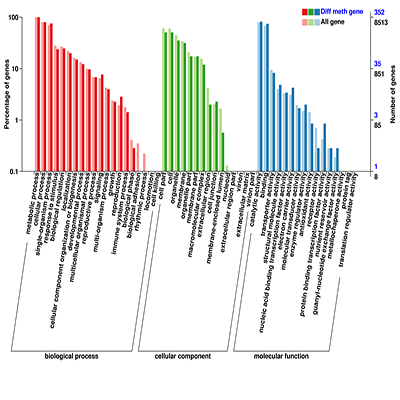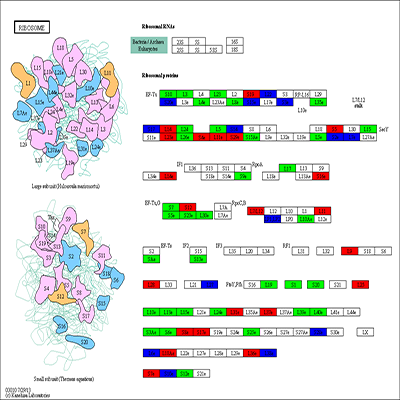DMR关联基因代谢通路分析

### 常见问题

1. 每个DMR区的片段长度不同是为什么？例如一个DMR长度将近1 kb，那这1 kb是怎么来的，这1 kb的任意一段是不是就不是DMR了？

2. 甲基化水平计算方法都有哪些？

1)单个位点甲基化水平：

2)区域甲基化水平计算方法1-Fraction of methylated cytosines

3)区域甲基化水平计算方法2-Mean methylation level

a)甲基化水平＝(2/2+3/3+20/21+4/30+4/25+3/17+3/20+6/7+4/6+5/5)/12
b)甲基化水平＝(1/5+1/3+3/12+40/41+29/31+30/32+77/87+45/47+4/12+1/7+2/10)/12
4)区域甲基化水平计算方法3-Weighted methylation level

a)甲基化水平＝(2+3+20+4+4+3+3+6+4+5)/(2+3+21+30+25+17+20+7+6+5+18+25)
b)甲基化水平=(1+1+3+40+29+30+77+45+4+1+2)/(5+3+12+16+41+31+32+87+47+12+7+10

### 相关文章

•Cancer Cell | 癌症全基因组甲基化研究
人类癌症表观基因组学很大程度上被重新制定，包括基因启动子区CpG岛的超甲基化和全基因组水平的低甲基化。CpG岛 […]
阅读更多